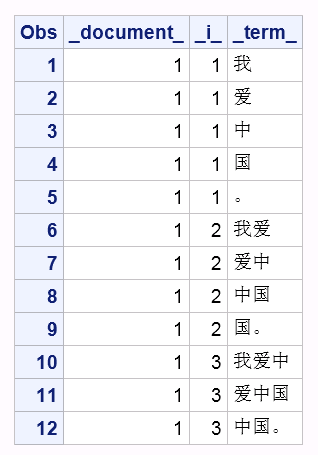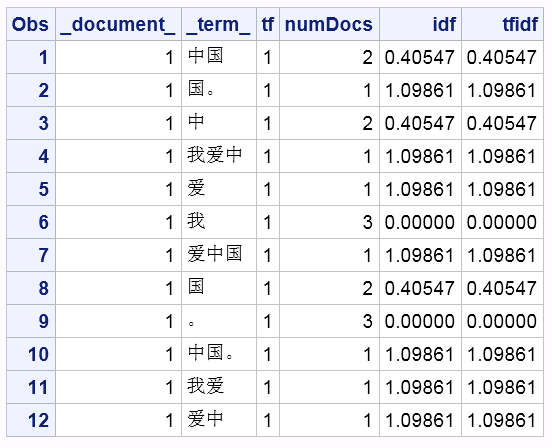SAS Visual Text Analytics provides dictionary-based and non-domain-specific tokenization functionality for Chinese documents, however sometimes you still want to get N-gram tokens. This can be especially helpful when the documents are domain-specific and most of the tokens are not included into the SAS-provided Chinese dictionary.

### What is an N-gram?

An N-gram is a sequence of N items from a given text with n representing any positive integer starting from 1. When n is 1, it refers to a unigram; when n is 2, it refers to a bigram; when n is 3, it refers to a trigram. For example, suppose we have a text in Chinese "我爱中国。", which means "I love China." Its N-gram sequence looks like the following:

 n Size N-gram Sequence 1 [我], [爱], [中], [国], [。] 2 [我爱], [爱中], [中国], [国。] 3 [我爱中], [爱中国], [中国。]

### How many N-gram tokens are in a given sentence?

If Token_Count_of_Sentence is number of words in a given sentence, then the number of N-grams would be:

`Count of N-grams = Token_Count_of_Sentence – ( n - 1 )`

The following table shows the N-gram token count of "我爱中国。" with different n sizes.

 n Size N-gram Sequence Token Count 1 [我], [爱], [中], [国], [。] 5 = 5- (1-1) 2 [我爱], [爱中], [中国], [国。] 4 = 5- (2-1) 3 [我爱中], [爱中国], [中国。] 3 = 5- (3-1)

In real actual language processing (NLP) tasks, we often want to get unigram, bigram and trigram together when we set N as 3. Similarly, when we set N as 4, we want to get unigram, bigram, trigram, and four-gram together.

N-gram theory is very simple and under some conditions it has big advantage over dictionary-based tokenization method, especially when the corpus you are working on has many vocabularies out of the dictionary or you don't have a dictionary at all.

### How to get N-grams with SAS?

SAS is a powerful programming language when you manipulate data. Below you'll find a program I wrote, using the DATA step to get N-grams.

```data data_test; infile cards dlm='|' missover; input _document_ text :\$100.; cards; 1|我爱中国。 ; run;   data NGRAMS; set data_test; _tmpStr_ = text; do while (klength(_tmpStr_)>0); _maxN_=min(klength(_tmpStr_), 3); do _i_=1 to _maxN_; _term_ = ksubstr(_tmpStr_, 1, _i_); output; end; if klength(_tmpStr_)>1 then _tmpStr_ = ksubstr(_tmpStr_, 2); else _tmpStr_ = ''; end; keep _document_ _term_ _i_; run;```

Let's see the SAS results.

```proc sort data=NGRAMS; by _document_ _i_; run;   proc print; run;```N-grams tokenization is the first step of NLP tasks. For most NLP tasks the second step is to calculate the term frequency–inverse document frequency (TF-IDF). Here's the approach:

`tfidf(t,d,D) = tf(t,d) * idf(t,D)`
`IDF(t) = log_e(total number of documents / number of documents that contain term t)`

Where t denotes the terms; d denotes each document; D denotes the collection of documents.

Suppose that you need to handle process lots of documents -- let me show you how to do it using SAS Viya. I used these four steps.

#### Step 1: Start CAS Server and create a CAS library.

```cas casauto host="host.example.com" port=5570; libname mycas cas;   <h4>Step 2: Load your data into CAS. </h4> Here to simply the code, I only tried 3 sentences for demo purpose. data mycas.data_test; infile cards dlm='|' missover; input _document_ fact :\$100.; cards; 1|我爱中国。 2|我是中国人。 3|我是山西人。 ; run;```

Once the data in loaded to CAS, you may run following code to check the column information and record count of your corpus.

```proc cas; table.columnInfo / table="data_test"; run;   table.recordCount / table="data_test"; run; quit;```

#### Step 3: Tokenize texts into N-grams

```%macro TextToNgram(dsin=, docvar=, textvar=, N=, dsout=); proc cas; loadactionset "dataStep"; dscode = "data &dsout; set &dsin; length _term_ varchar(&N); _tmpStr_ = &textvar; do while (klength(_tmpStr_)>0); _maxN_=min(klength(_tmpStr_), &N); do _i_=1 to _maxN_; _term_ = ksubstr(_tmpStr_, 1, _i_); output; end; if klength(_tmpStr_)>1 then _tmpStr_ = ksubstr(_tmpStr_, 2); else _tmpStr_ = ''; end; keep &docvar _term_; run;"; runCode code = dscode; run; quit; %mend TextToNgram;   %TextToNgram(dsin=data_test, docvar=_document_, textvar=text, N=3, dsout=NGRAMS);```

#### Step 4: Calculate TF-IDF.

```%macro NgramTfidfCount(dsin=, docvar=, termvar=, dsout=); proc cas; simple.groupBy / table={name="&dsin"} inputs={"&docvar", "&termvar"} aggregator="n" casout={name="NGRAMS_Count", replace=true}; run; quit;   proc cas; simple.groupBy / table={name="&dsin"} inputs={"&docvar", "&termvar"} casout={name="term_doc_nodup", replace=true}; run;   simple.groupBy / table={name="term_doc_nodup"} inputs={"&docvar"} casout={name="doc_nodup", replace=true}; run; numRows result=r/ table={name="doc_nodup"}; totalDocs = r.numrows; run;   simple.groupBy / table={name="term_doc_nodup"} inputs={"&termvar"} aggregator="n" casout={name="term_numdocs", replace=true}; run;   mergePgm = "data &dsout;" || "merge NGRAMS_Count(keep=&docvar &termvar _score_ rename=(_score_=tf)) term_numdocs(keep=&termvar _score_ rename=(_score_=numDocs));" || "by &termvar;" || "idf=log("||totalDocs||"/numDocs);" || "tfidf=tf*idf;" || "run;"; print mergePgm; dataStep.runCode / code=mergePgm; run; quit; %mend NgramTfidfCount;   %NgramTfidfCount(dsin=NGRAMS, docvar=_document_, termvar=_term_, dsout=NGRAMS_TFIDF);```

Now let's see the TFIDF result of the first sentence.

```proc print data=sascas1.NGRAMS_TFIDF; where _document_=1; run;```These N-gram methods are not designed only for Chinese documents; and documents in any language can be tokenized with this method. However, the tokenization granularity of English documents is different from Chinese documents, which is word-based rather than character-based. To handle English documents, you only need to make small changes to my code.

ShareDirector Analytics Development Groups, SAS Beijing R&D

Emily Gao manages two product lines: Decision Manager and Text Analytics. She received her data mining masters degree in 2002, and has been with SAS for 15-years.

### 1 Comment

1.Thank you for your article over N-GRAM and more over your way of explanation. Currently, i'm working on sentimental analysis of Review data (Amazon data) on SAS EG. I have completed the process of eliminating stoplist word from raw data and doing N-gram process on data, i did TRI-gram on my data but i'm confuse how to do next step for that output data from n-gram process, my aim is to calculate only frequency(term frequency) of neutral, positive and negative word. so could you please help me with any article or any example.

Regards,
Ranjan

This site uses Akismet to reduce spam. Learn how your comment data is processed.